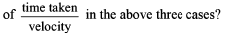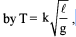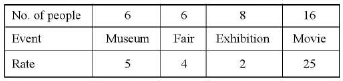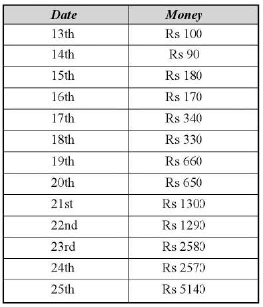Courses

# Test: Ratio And Proportion- 5

## 14 Questions MCQ Test Quantitative Aptitude (Quant) | Test: Ratio And Proportion- 5

Description
This mock test of Test: Ratio And Proportion- 5 for Quant helps you for every Quant entrance exam. This contains 14 Multiple Choice Questions for Quant Test: Ratio And Proportion- 5 (mcq) to study with solutions a complete question bank. The solved questions answers in this Test: Ratio And Proportion- 5 quiz give you a good mix of easy questions and tough questions. Quant students definitely take this Test: Ratio And Proportion- 5 exercise for a better result in the exam. You can find other Test: Ratio And Proportion- 5 extra questions, long questions & short questions for Quant on EduRev as well by searching above.
QUESTION: 1

### In an election, each voter may vote for two candidates. Half of the voters vote for A, but divide their votes between B, C and D in the ratio of 3 : 2 : 1. Of the remaining, half vote for B and divides their votes between C and D in the ratio of 2 : 1. Of the remaining, half vote for C and D and the remaining 840 do not vote for anyone. How many votes were received by A, B, C and D respectively?

Solution:

Best way of solving this question is—Go through the options.

QUESTION: 2

### In a forest reserve, the ratio of the number of deers, bear and fox is 3 : 7 : 5. If the difference between the number of deers and bears is a multiple of 3 as well as 7, what is the minimum number of animals in the park?

Solution:

No. of deers, bears and foxes = 3x : 7x : 5x Difference between the no. of deers and bears = 4x Now, 4x has to be a multiple of 21. So, x has to be a multiple of 21.
So, Total number of animals = 15x =15 x 21 = 315

QUESTION: 3

### Shalaj and Associates, a shoe-manufacturing company, has three machines producing shoes. If machine X produces l/4th as many as machine Y produces in the same time, and machine Y produces twice as many as machine Z produces in the same time, then during a fixed time, what fraction of the total number of shoes is produced by machine Z?

Solution:

Assume that Y produces 4P units in unit time. Then X and Z produce P and 2P units in the same time. So, Total shoes produced = 7P, Fraction of the total number of shoes produced by machine Z = 2/7

QUESTION: 4

The inhabitants of Hawaii Island are taxed in a peculiar manner. They have to pay a fixed sum irrespective of their income strata. In addition to this they have to pay an amount which is proportional to the excess of their salary over Rs 50,000. Mr Kalyan pays a total tax of Rs 6,200 when his annual salary is Rs 6,0000 and his wife Mrs. Kalyan pays a total tax of Rs 7,700 when her annual salary is Rs 75,000. What is the yearly salary of Mr Ajay who pays an annual tax of Rs 8,200?

Solution:

Tax = Fixed + Variable

Since Mr Kalyan pays Rs 6200 and Mrs Kalyan pays Rs 7700, so it can be inferred that for Rs 10,000 (Difference between their salaries), one has to pay Rs 1500 as the variable tax. So, Fixed component of tax = Rs 5200. The point worth to be noted here is the fact that tax is to be paid over an above Rs 50,000. So, the variable tax percentage above Rs 50,000 = 10%. Now the answer can be calculated using options.

QUESTION: 5

The total profit made by running a train between New Delhi to Lucknow consists of two parts: (i) a constant amount of Rs 24 lakh per trip and (ii) it varies as the square of the number of coaches attached to the engine that amounts to Rs 7P2, where P is the number of coaches attached to the engine in the trip. If the average profit per coach per trip should not fall below Rs 169 lakh, then what is the minimum number of coaches that have to be attached to the engine?

Solution:

otal profit = Rs 24 lakh per trip + Rs 7P2, where P is the number of coaches.
Now go through the options.

QUESTION: 6

The average age of the students in a class of 50 is 13. The weight of each student is directly proportional to the height. A 165 cm tall student has a weight of 33 kg. The average weight of the class is:

Solution:

The correct option is Option B.

We know, weight is directly proportional to height.

Also, height is directly proportional to age.

So, we can say that weight is directly proportional to age.

Given, The average age is 13.

An 11 year old student weighs 33 kg.

So, a 13 year old student would weigh

= 33 × (13/11)

= 39 kg

QUESTION: 7

The speed of man while walking, running and driving are in the ratio of 1:3:9. If he travels equal distance while walking, running and driving, what is the ratioSolution:

Assume the distance = 9 units. So, the ratio of the time taken = 9:3:1 So, the answer = 9/1:3/3:1/9

QUESTION: 8

The time period of oscillation T for a pendulum is givenwhere i is the length o f the pendulum and g is the acceleration due to gravity, ‘k ’ is any const. If ‘k ’ and ‘g ’ rem ain unchanged under any condition, what should be the percentage change in l such that the time period T increases by 10%?

Solution:

Explanation : T = 2π * (√l/g)

T increases by 10%, new time period 0.1T

(1T+0.1T) = 2π * (√l/g)

1.1T = 2π * (√l/g)

i.e 21% increase in the length of the pendulum.

QUESTION: 9

In the famous Hawthorne studies by Elton Mayo, employees of the organization were given the options of going to several places for entertainment. One-seventh of the employees preferred to go to the polo ground, a number equal to the square root of the remaining employees went to the museum. One-fifth of the remaining employees went to the fair. One-third of the remaining to the science exhibition and the rest to watch movie ASHOKA. which was running house full in the nearby theatre. The total number of employees who went to the polo ground and the museum is twice that of the employees who went to the fair.
Q.The entry tickets for museum, fair, exhibition and the movie were Rs 5, Rs 4, Rs 2 and Rs 25 respectively. How much money did the employees pay for all these on that Sunday?

Solution:QUESTION: 10

Read the passage below and solve the questions based on it.
On 13th of November, I kept some money in a magical purse in which on even every alternate day starting from the 13th, money increases with respect to the closing amount on the evening of the previous day, that the ratio of money becomes 2 : 1 but on every alternate day starting from the 14th, the money decreases by Rs 10. when compared to the closing amount on the evening of the previous day I had Rs 500 on the 19th and the magical purse kept on multiplying money for me till the 25th of that November.
Q. How much money was there on the 22nd of November?

Solution:

It is Rs 970 on 22nd.

QUESTION: 11

Read the passage below and solve the questions based on it.
On 13th of November, I kept some money in a magical purse in which on even every alternate day starting from the 13th, money increases with respect to the closing amount on the evening of the previous day, that the ratio of money becomes 2 : I but on every alternate day starting from the 14th, the money decreases by Rs 10. when compared to the closing amount on the evening of the previous day I had Rs 500 on the 19th and the magical purse kept on multiplying money for me till the 25th of that November.
Q. Had I started with Rs 100, how much more/less money would I have received than I am receiving now?

Solution:

The new table would have been like this:Now it can be calculated.

QUESTION: 12

Read the passage below and solve the questions based on it.
In a class, every student plays exactly one of the following games—squash, table tennis, hockey, handball, cricket. Exactly 1/ 12th of the girls and 1/8th of the boys play- squash. 1/15th of the girls and 1/6th of the boys play hockey, 1/4th of the girls and 1/12th of the boys play handball. 1/5th of the girls and 3/8th of the boys plays table tennis and the remaining boys and girls play cricket. The total strength of the class is less than 120
Q. How many girls are there in the class?

Solution:

Whatever is the total number of boys, it should be divisibie by 8, 6, 12 and total number of girls should be divisible by 12, 15 and 4, Now use options.

QUESTION: 13

Read the passage below and solve the questions based on it.
In a class, every student plays exactly one of the following games—squash, table tennis, hockey, handball, cricket. Exactly 1/ 12th of the girls and 1/8th of the boys play- squash. 1/15th of the girls and 1/6th of the boys play hockey, 1/4th of the girls and 1/12th of the boys play handball. 1/5th of the girls and 3/8th of the boys plays table tennis and the remaining boys and girls play cricket. The total strength of the class is less than 120
Q. Which of the following cannot be the ratio of boys and girls in the class?

Solution:

Whatever is the total number of boys, it should be divisibie by 8, 6 and 12 and total number of girls should be divisible by 12, 15 and 4, Now use options.

QUESTION: 14

The expenses of a hostel are partly fixed and partly variable with the number of boarders. When there are 50 boarders the charge is Rs. 48 per head and when there are 30 boarders the charge is Rs. 60 per head. Find the charges when there are 120 boarders?

Solution:

F = Fixed Cost
K = variable cost
B= No of boarders
E= Total Expenditure
E= F + KB
2400 = F + K50
1800 = F + K30
K= 30
F = 900
When there are 120 boarders the charge will be
E = 900 + 30(120)
E= 4500
Charge per boarder = 4500/120 = 37.5